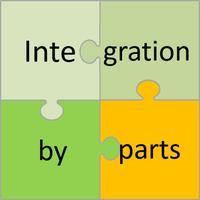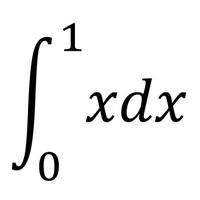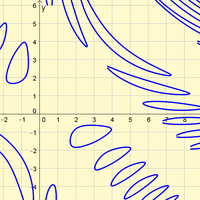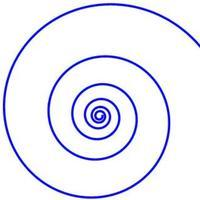## Hot pages

•### Integration by PartsCalculus

Integration by parts is a method of integration that we use to integrate the product (usually !) of two functions. The aim is to change this product into another one that is easier to integrate. Although the formula looks quite odd at first glance, the tec

•### Integration by Substitution HLCalculus

Integration by substitution or U-substitution is a method that will help you integrate many different functions. By changing the variable of the integrand, we can make an apparently difficult problem into a much simpler one. The challenge is recognising wh

•### Definite IntegrationCalculus

Integration is sometimes called antidifferentiation, as it is the opposite process of differentiation. When we find an indefinite integral we find a function with an abritrary constant, C. When we find a definite integral, we find a numerical value.

•### Graphs of Implicit EquationsCalculus

Implicit Equations are often used to represent relations that cannot be expressed as functions (one to many relations or many to many relations). These often have really interesting graphs. This page will allow to explore some of these graphs and consider

•### Indices and LogarithmsAlgebra

Logarithms are useful for solving problems involving indices (or exponents). In fact, logarithms are just indices in disguise! The definition of a logarithm helps you to see its equivalence with indices